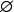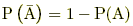index: click on a letter A B C D E F G H I J K L M N O P Q R S T U V W X Y Z A to Z index index: subject areas numbers & symbols sets, logic, proofs geometry algebra trigonometry advanced algebra & pre-calculus calculus advanced topics probability & statistics real world applications multimedia entrieswww.mathwords.com about mathwords website feedback

Probability

The likelihood of the occurrence of an event. The probability of event A is written P(A). Probabilities are always numbers between 0 and 1, inclusive.

 The four basic rules of probability: 1. For any event A, 0 ≤ P(A) ≤ 1. 2. P(impossible event) = 0. Also written P(empty set) = 0 or P() = 0. 3. P(sure event) = 1. Also written P(S) = 1, where S is the sample space. 4. P(not A) = 1 – P(A). Also written P(complement of A) = 1 – P(A)   or   P(AC) = 1 – P(A)   or. If all outcomes of an experiment are equally likely, then